# Kaiser window

The Kaiser window coefficients are given by the following formula

$$a(k)=\frac{I_0(\pi\,\alpha \sqrt{1-(\frac{2k}{N-1}-1)^2}\,)}{I_0(\pi\,\alpha)}$$

where N is the length of the filter, k = 0, 1, …, N – 1, α is any real number, and I0 is the zero order modified Bessel function of the first kind.

## Computing the Kaiser window

The modified Bessel function of the first kind (the hyperbolic Bessel function) is defined as follows.

$$I_{\beta}(x)=\sum_{n=0}^{\infty}\frac{1}{n!\,\Gamma(n+\beta+1)}(\frac{x}{2})^{2n+\beta}$$

where β is the order of the function and Γ is the generalized factorial.

Note that for any positive integer n, Γ(n) = (n – 1)!. Then at β = 0, Γ(n + β + 1) = (n + 0 + 1 – 1)! = n!. This means that the zero order modified Bessel function of the first kind is simply

$$I_0(x)=\sum_{n=0}^{\infty}(\frac{1}{n!})^2(\frac{x}{2})^{2n}$$

The sum converges and we can be approximate for any x, if we take the first few terms, say

$$I_0(x)=\sum_{n=0}^{M}(\frac{1}{n!})^2(\frac{x}{2})^{2n}$$

The Kaiser window itself, if we rewrite the numerator and denominator as per the discussion above, can be written as follows.

$$a(k)=\frac{\sum_{n=0}^{\infty}(\frac{1}{n!})^2(\frac{\pi\, \alpha \sqrt{1-(\frac{2k}{N-1}-1)^2}}{2})^{2n}}{\sum_{n=0}^{\infty}(\frac{1}{n!})^2(\frac{\pi\, \alpha}{2})^{2n}}$$

As before, both sums converge and can be approximated by taking the first few terms.

## An example Kaiser window

Consider a finite impulse response (FIR) low pass filter of length N = 201. The following is the Kaiser window with α = 3 and M = 4.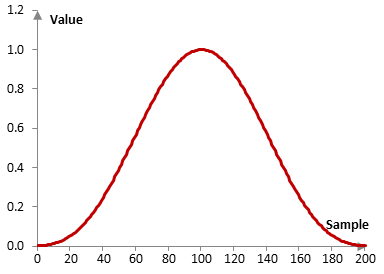Given a sampling frequency of 2000 Hz and a filter cutoff frequency of 40 Hz, the impulse response of the filter with a rectangular window (with no window) and with the Kaiser window is as follows.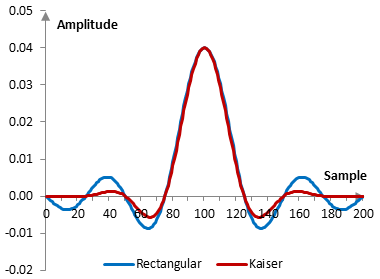The magnitude response of the same filter is shown on the graph below.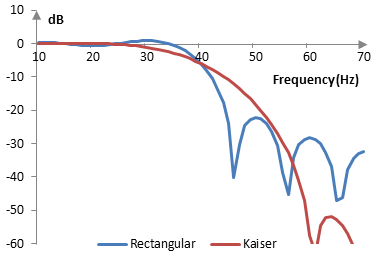As the absolute value of α increases, the windowed filter produces a larger transition band, but better stop band attenuation. As |α| decreases, the filter has a smaller transition band and worse stop band attenuation. At α = 0 the Kaiser window becomes a rectangular window.

The Kaiser window with N = 201 for three different values of α (0.5, 1, and 5) is shown below.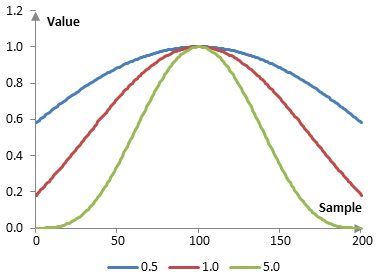The following are the magnitude responses of these windows, applied to a filter with a cutoff frequency of 40 Hz, given a sampling frequency of 2000 Hz.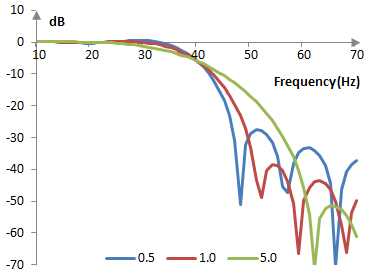## Measures for the Kaiser window

The following is a comparison of the discrete Fourier transform of the Kaiser window (α = 1.0) and the rectangular window.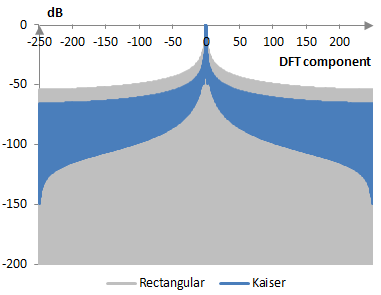The Kaiser window measures are as follows.

 α 0.5 1.0 5.0 Coherent gain 0.85 0.67 0.42 Equivalent noise bandwidth 1.02 1.15 1.76 Processing gain -0.10 dB -0.62 dB -2.44 dB Scalloping loss -3.31 dB -2.42 dB -1.05 dB Worst case processing loss -3.41 dB -3.04 dB -3.49 dB Highest sidelobe level -16.6 dB -24.6 dB -38.6 dB Sidelobe falloff -6.4 dB / octave, -21.2 dB / decade -7.3 dB / octave, -24.2 dB / decade -15.5 dB / octave, -51.6 dB / decade Main lobe is -3 dB 0.96 bins 1.10 bins 1.68 bins Main lobe is -6 dB 1.30 bins 1.52 bins 2.34 bins Overlap correlation at 50% overlap 0.479 0.370 0.080 Amplitude flatness at 50% overlap 0.895 0.829 0.691 Overlap correlation at 75% overlap 0.794 0.783 0.559 Amplitude flatness at 75% overlap 0.974 0.958 0.991

Window

### Filtered HTML

• Freelinking helps you easily create HTML links. Links take the form of [[indicator:target|Title]]. By default (no indicator): Click to view a local node.